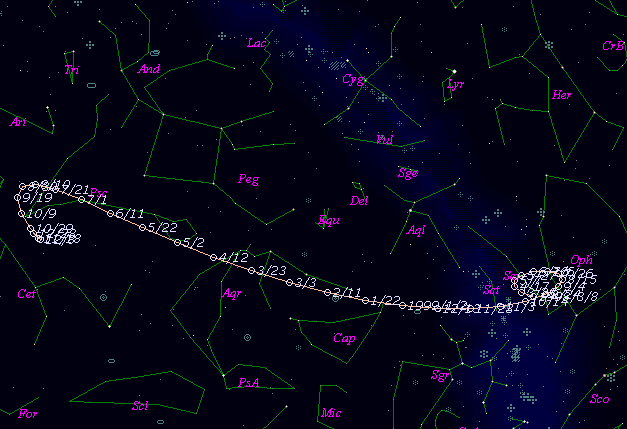# \$B3k(B-\$B]jWB@1(B

142P/Ge-Wang (1999)###\$B%W%m%U%#!<%k(B

 \$BId9f(B 142P/1999 R2 \$B8!=PF|(B 1999\$BG/(B9\$B7n(B15\$BF|(B \$B8!=P8wEY(B 20.3\$BEy(B \$B8!=P J. V. Scotti (Kitt Peak)

###\$B50F;MWAG(B

```   The following improved orbital elements by Kenji Muraoka, are
from 18 observations 1988 Oct. 11 to 1999 Sept.16, perturbations
by 9 Plantes, Moon and 5 minor planets were taken into account.
The mean residual is +/- 0.87 arc seconds.

Epoch  =  1999 July  1.0  TT       JDT = 2451360.5
T  =  1999 June 21.50534       +/- 0.01832 (m.e.) TT
Peri. =  177.39048                +/- 0.00670
Node  =  177.13643                +/- 0.00258   (2000.0)
Incl. =   12.17161                +/- 0.00092
q  =    2.4961208              +/- 0.0002460 AU
e  =    0.5003733              +/- 0.0000489
a  =    4.9959717              +/- 0.0001388 AU
n  =    0.08826207             +/- 0.00000368
P  =   11.167                  +/- 0.0004655  years
```

###\$B@1?^(B###\$B8wEYJQ2=(B

```        m1 = 12.5 + 5 log\$B&\$(B + 15.0 log r
```##### \$B50F;MWAG\$OB<2,7r<#;a\$N7W;;\$K\$h\$k\$b\$N\$G\$9!#(B \$B@1?^\$O(B StellaNavigator Ver.2.0 for Windows (\$B%"%9%H%m%"!<%D(B \$BJTCx(B / \$B%"%9%-!<=PHG6I4)(B) \$B\$G:n@.\$7\$?\$b\$N\$G\$9!#(B \$B8wEY%0%i%U\$O(BComet for Windows\$B\$G:n@.\$7\$?\$b\$N\$G\$9!#(B